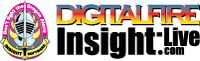•The secret to cool bodies and glazes is a lot of testing.
•The secret to know what to test is material and chemistry knowledge.
•The secret to learning from testing is documentation.
•The place to test, do the chemistry and document is an account at https://insight-live.com
•The place to get the knowledge is https://digitalfire.com

Sign-up at https://insight-live.com today.

# CP-7 Ball Clay

Alternate Names: CP #7

 Oxide Analysis Formula CaO 0.30% 0.019 MgO 0.10% 0.009 K2O 0.14% 0.005 Na2O 0.05% 0.003 TiO2 1.60% 0.071 Al2O3 28.67% 1.000 SiO2 54.89% 3.249 Fe2O3 1.00% 0.022 LOI 12.65 Oxide Weight 308.63 Formula Weight 353.33
 PCE - Pyrometric Cone Equivalent 33

## XML for Import into INSIGHT

<?xml version="1.0" encoding="UTF-8"?> <material name="CP-7 Ball Clay" descrip="" searchkey="CP #7" loi="0.00" casnumber="70694-09-6"> <oxides> <oxide symbol="CaO" name="Calcium Oxide, Calcia" status="" percent="0.300" tolerance=""/> <oxide symbol="MgO" name="Magnesium Oxide, Magnesia" status="" percent="0.100" tolerance=""/> <oxide symbol="K2O" name="Potassium Oxide" status="" percent="0.140" tolerance=""/> <oxide symbol="Na2O" name="Sodium Oxide, Soda" status="" percent="0.050" tolerance=""/> <oxide symbol="TiO2" name="Titanium Dioxide, Titania" status="" percent="1.600" tolerance=""/> <oxide symbol="Al2O3" name="Aluminum Oxide, Alumina" status="" percent="28.670" tolerance=""/> <oxide symbol="SiO2" name="Silicon Dioxide, Silica" status="" percent="54.890" tolerance=""/> <oxide symbol="Fe2O3" name="Iron Oxide, Ferric Oxide" status="" percent="1.000" tolerance=""/> </oxides> <volatiles> <volatile symbol="LOI" name="Loss on Ignition" percent="12.650" tolerance=""/> </volatiles> </material>